# Bihar Board Class 12 Physics Solutions Chapter 15 Communication Systems

Bihar Board Class 12 Physics Solutions Chapter 15 Communication Systems Textbook Questions and Answers, Additional Important Questions, Notes.

## BSEB Bihar Board Class 12 Physics Solutions Chapter 15 Communication Systems

### Bihar Board Class 12 Physics Communication Systems Textbook Questions and Answers

Question 1.
Which of the following frequencies will be suitable for beyond-the-horizon communication using sky waves?
(a) 10 kHz
(b) 10 MHz
(c) 1 GHz
(d) 1000 GHz
(b) i.e., 10 MHz.
10 kHz frequencies cannot be radiated because of the antenna size required for their propagation while the frequencies 1 GHz and 1000 GHz will penetrate and thus cannot reach to the receiver beyond the horizon.

Question 2.
Frequencies in the UHF range normally propagate by means of:
(a) Ground waves
(b) Sky waves
(c) Surface waves
(d) Space waves.
(d) i.e., space waves i.e., propagation of e.m. waves in ultra ugh frequency range is possible by space waves.Question 3.
Digital signals
(i) do not provide a continuous set of values
(ii) represent values as discrete steps
(iii) can utilize binary system, and
(iv) can utilize decimal as well as binary systems.
Which of the above statements are true?
(a) (i) and (ii) only
(b) (ii) and (iii) only
(c) (i), (ii) and (iii) but not (iv)
(d) All of (i), (ii), (iii) and (iv).
(c) Digital signals represented by 0 and 1 don’t provide continuous set of values, (ii) they represent values in discrete stps. (iii) utilize only binary system.

Question 4.
Is it necessary for a transmitting antenna to be at the same height as that of the receiving antenna for line-of-sight communication? A TV transmitting antenna is 81 m tall. How much service area it can cover if the receiving antenna is at the ground level?
No, it is not necessary for a transmitting antenna to be at the same height as that of the receiving antenna for a line of sight communication. :
Here, h = height of TV antenna = 81 m.
R = radius of earth = 6400 km = 6.4 × 106 m.
Let d be the radius of the service area it can cover i.e., the radius of the circle within which the transmission can be observed.
Using the relation, d = $$\sqrt{2 \mathrm{hR}}$$ , we get
d = $$\sqrt{2 \times 81 \times 6.4 \times 10^{6}}$$m
= 9 × 8 × 103 × $$\sqrt{0.2}$$ m
Let A = service area in which transmission can be received from this antenna = ?
∴ Using the formula,
A = πd2, we get
A = π × $$\left(72 \times 10^{3} \times \sqrt{0.2}\right)^{2}$$
= π × 81 × 64 × 106 × 0.2 m2
= 3258 × 106  × 0.2 m2 = 3258 km2.

Question 5.
A carrier wave of peak voltage 12 V is used to transmit a message signal. What should be the peak voltage of the modulating signal in order to have a modulation index of 75%?
Here, Ec = amplitude of the carrier = 12 V.
Em = peak voltage i.e., amplitude of the modulating signal = ?
ma = modulation index = 75% = $$\frac{75}{100}$$
Using the relation, ma = $$\frac{\mathrm{E}_{\mathrm{m}}}{\mathrm{E}_{\mathrm{c}}}$$, we get
Em= ma × Ec
= 0. 75 × 12 = 9 V.

Question 6.
A modulating signal is a square wave as shown in fig.:The carrier wave is given by c(t) = 2 sin (8 πt) volts.
(i) Sketch the amplitude modulated waveform
(ii) What is the modulation index?
(i) The sketch of the amplitude modulated waveform is shown below: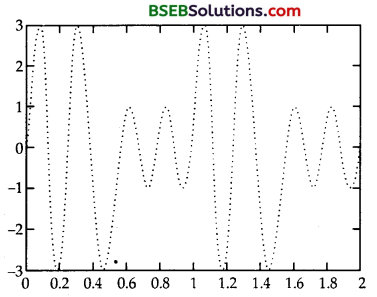(ii) Here, the carrier wave
C(t) = 2 sin (8 πt) (volts)
Ec= amplitude of carrier wave = 2V
Em= amplitude of the modulating signal = IV
ma= modulation index of A.M.
Waveform = ?
We know that, ma = $$\frac{\mathrm{E}_{\mathrm{m}}}{\mathrm{E}_{\mathrm{c}}}$$ = $$\frac{1}{2}$$ = $$\frac{1}{2}$$ = 0.5Question 7.
For an amplitude modulated wave, the maximum amplitude is found to be 10V while the minimum amplitude is found 1 be 2V. Determine the modulation index, μ.
What would be the value of μ if the minimum amplitude is zero volt?
Modulation index μ = $$\frac{\text { amplitude of modulating wave }}{\text { amplitude of unmodulated }}$$
μ = $$\frac{\mathrm{V}_{\mathrm{m}}}{\mathrm{V}_{\mathrm{c}}}$$
Modulating index increases as modulating frequency fm decrease As mf is the ratio of two frequencies, it is measured in radians.Question 8.
Due to economic reasons, only the upper sideband of an AM wave is transmitted, but at the receiving station, there is a facility for generating the carrier. Show that if a device is available which can multiply two signals, then it is possible to recover the modulating sign it at the receiver station.
Let ec and em be the instantaneous.
Voltages or carried and modulating wavesterpngtively and ar, given by
ec = vc sin wct ……….. (1)
em = vm sin wmt ……….. (2)
For modulated wave
e = V sin wct
Where V = amplitude of modulated Wave
\begin{aligned} &=\left(v_{c}+e_{m}\right) \sin w_{c} t \\ &=\left(v_{c}+e_{m} \sin w_{m} t\right) \sin w_{c} t \\ &=v_{c} \sin w_{c} t+e_{m} \sin w_{m} t \sin w_{c} t \\ &=v_{c} \sin w_{c} t+m v_{c} \sin w_{m} t \sin w_{c} t \end{aligned}
(∵$$\mathrm{m}=\frac{\mathrm{V}_{m}}{\mathrm{~V}_{\mathrm{c}}}$$)
Vc sin wct m Vc $$\left[\frac{1}{2} \cos \left(w_{c}-w_{m}\right) t-\frac{1}{2} \cos \left(w_{c}+w_{m}\right) t\right]$$
V = Vc sin wct + $$\frac{\mathrm{mv}_{\mathrm{c}}}{2}$$ cos $$\left(w_{c}-w_{m}\right) t-\frac{m v_{c}}{2} \cos \left(w_{c}+w_{m}\right) t$$ ……….. (3)
Eq (3) represents the simplitude modulated wave recived at the receiving station from the transmitting station. If only upper sideband
of AM wave is transmitted, then V = sin wct – $$\frac{\mathrm{mv}_{\mathrm{c}}}{2}$$ cos (Wc + Wm)t
To recover the modulating signal at the receiving station, the modulated wave is fet to a circuit or a device known as detector It demolutates the modulating signal from the modulated carrier wave i.e, it recovers the modulated signal from the molulated carrier wave i.e. rectifying the input signal. Thus either the top or bottom half of the wave is removed, a suitable filter removes the raido frequency of the rectified wave and a signal is produced which allows the envelope of one-half of the wave. Here, upper side band is transmitted so the out
put follows this half and is of the form of the modulating wave. The waveforms are as shown below.Aliter:
Let A’ = Ac cos Wc)t se the carrier signal.
Let, for simplicity, the received signal be
A1cos (wc> + wa)t
the carrier Ac cos wct is available at the receiving station. By multiplying the two signals, we get
A1 Ac (wc + wa)t cos wct
= $$\frac{A_{1} A_{c}}{2}$$ [cos (2wc> + wm) t + cos wmt]
If this signal is passed through a low-pass filter, we can record the modulating signal $$\frac{A_{1} A_{c}}{2}$$ cos wmt.

### Bihar Board Class 12 Physics Communication Systems Additional Important Questions and Answers

Question 1.
Define Communication.
It is defined as the process of sending and receiving of information with the help of a suitable communication system and transmission medium.

Question 2.
Define modulation.
It is defined as the process of producing a high frequency wave some characteristic of which varies as a function of the instantaneous value of the audio signal.Question 3.
Define the following :
(a) Modulating wave.
(b) Carrier wave.
(c) Modulated wave.
(a) It is defined as the audio signal to be modulated. It is mixed with high frequency wave. It is also called base band signal.
(b) It is defined as the high frequency wave.
(c) It is defined as the resultant wave produced due to the superposition of modulating wave on the carrier wave.

Question 4.
Define the following:
(a) Amplitude modulation.
(b) frequency modulation.
(a) It is defined as the process of producing a modulated wave having the same frequency as that of the carrier wave but its amplitude is modified in accordance with that of the modulating wave. It is shown below :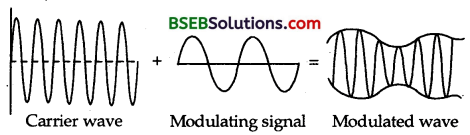(b) It is defined as the process of producing a modulated wave having amplitude same as that of the carrier wave but its frequency is modified in accordance with the amplitude of modulating wave.

Question 5.
Define a transducer.
It is defined as a device which converts energy from one form to another, e.g., microphone which converts sound signal into electrical signal.

Question 6.
Define modulation index. What is its importance?
It is defined as the degree to which the carrier wave is modulated. It helps us in determining the strength and quality of the transmitted signal. Higher the modulation index; stronger and more clear will be the reception. Thus it helps to know the nature of the signal received.

Question 7.
Define frequency deviation.
It is defined as the maximum swing of the frequency of the modulated wave from the carrier frequency.

Question 8.
Define demodulation.
It is defined as the process of separating out the modulating wave from the modulated wave.

Question 9.
Define the following:
(a) noise
(b) pulse amplitude modulation
(c) quantisation.
(a) It is defined as the undesired electrical signal.
(b) It is defined as the process in which amplitude of the pulses is changed in accordance with the modulating signal.
(c) It is defined as the process of dividing the maximum value of the analog voltage signal into a fixed number of levels so as to convert a pulse amplitude modulated signal into a binary code.

Question 10.
Define Fax.
It is defined as the electronic transmission of a document at a distant place through telephone line.Question 11.
Define a passive satellite.
It is defined as a satellite used only for reflecting the signals back to the earth stations. It does not have equipments on it so as to amplify the signal received from earth.

Question 12.
State two factors by which the range of transmission of signals by a TV tower can be increased?
It can be done by :
(i) Increasing the height of the tower.
(ii) Increasing the height of the receiving antenna so that it may directly intercept the signal from the transmitting antenna.

Question 13.
Define a modem.
It is defined as a device that performs the functions of both modulator and demodulator. It works as a modulator in the transmitting mode while as a demodulator in the receiving mode.

Question 14.
Define ground waves.
They are defined as the amplitude modulated radio waves travelling directly from one point to another following the surface of earth. They have frequency upto 1500 kHz.

Question 15.
Define Sky waves. Give their frequency range.
They are defined as the amplitude modulated radio waves received on earth after reflection from ionosphere. They have frequency range from 1500 kHz to 40 MHz.

Question 16.
Define transmission medium.
It is defined as a link that transfers information from the information source to the destination.

Question 17.
Name the various transmission media used in communication systems.
The following are the various transmission media used in communication systems:

1. Coaxial cables
4. Parallel-wire lines.

Question 18.
What is a Coaxial Cable?
It consists of a central copper wire surrounded by a PVC insulation over which a sleeve of copper mesh is placed and it is further covered with an outer shield of thick PVC material.

Question 19.
What is the advantage of using coaxial cable for transmitting a signal in place of 2 wire line?
The copper mesh surrounding the central copper wire makes it immune to the external electrical disturbances.

Question 20.
What do you understand by the form ‘switching circuit’ in a telephone system?
All the telephones in a locality are connected to the telephone exchange. When a calling subscriber dials in the called subscriber, the switching circuit interconnects the two.Question 21.
What is an optical fibre?
An optical fibre consists of a glass core surrounded by a glass coating of comparatively lesser refractive index. It is used to transmit optical signals from one place to another place.

Question 22.
It is defined as a glass coating surrounding the glass core in an optical fibre. Its refractive index is little lesser than that of the glasscore.

Question 23.
Define light modulation.
It is defined as the process of modulating a light beam from an optical source in accordance with the information signal.

Question 24.
Define direct intensity modulation.
It is defined as the process of light modulation in which the intensity of light is varied in accordance with the message signed in a direct manner by the optical source corresponding to the message signal.

Question 25.
Why is the transmission of signals using ground waves restricted upto a frequency of 1500 kHz?
It is because on further increasing the frequency of the signal, the absorption of the signal from the ground increases.

Question 26.
Define an active satellite.
It is a satellite that is equipped with the electronic devices so as to receive the signal from the earth station and then retransmit it back to earth after processing and amplifying it. It carries different types of sensors, e.g. Camera, 1 R scanner, microwave scanner etc. These transmit information in the form of data and pictures to the earth stations.

Question 27.
Why is it necessary to use satellites for long distance TV transmission?
It is necessary to use satellites for long distances TV transmission because TV signals are not reflected by the ionosphere and hence they are reflected back to the earth by making use of artificial satellites.

Question 28.
Why high frequency carrier waves are employed for transmission of audio signals?
High frequency carrier waves are employed for transmission of audio signals becuase these high frequency carrier waves travel * through space or medium with the speed of light and they are not obstructed by earth’s atmosphere.

Question 29.
Define Channel.
It is usually referred to the frequency range allocated to a particular service or transmission.

Question 30.
Give the basic difference between an analog communication system and a digital communication system.
An analog communication system makes use of analog signals that vary continuously with time while a digital system makes use of a digital signal which has only two values of the signal either high or low.Question 31.
How would you express amplitude, frequency and phase modulation?
We know that the equation of a carrier wave is expressed as:
E = E0 sin (ωt + Φ)
where E = instantaneous voltage at a time t, to is angular frequency, Φ = phase w.r.t. some reference, E0 = amplitude
So by varying E0, ω and Φ, we get amplitude, frequency and phase modulation.

Question 32.
Why is a F.M. signal less susceptible to noise than an AM signal?
in FM transmission, message is in the form of frequency variation of carrier waves. During modulation, noise gets amplitude modulated, changing the amplitude of carrier waves, thus message signal in the form of frequency variations is not affected. This is why FM signal is less susceptible to noise than an AM signal.

Question 33.
Define the following:
(a) Sampling
(b) Telemetry.
(a) It is the process of generating pulses of zero width i.e., duration and of amplitude equal to the instantaneous amplitude of the analog modulating signal.
(b) It is a system of transmitting data from a space craft to earth using radio waves.

Question 34.
Why does the electrical conductivity of earth’s atmosphere increases with altitude?
We know that the atmospheric pressure decreases as the height increases. The high energy particles liker y and cosmic rays coming from outer space cause ionisation of atoms of the gases present in earth’s atmosphere. Their ionising power decreases rapidly as they approach earth’s surface due to increase in the number of collisions with the gasous atoms.

Question 35.
Why the sky waves are not used in T.V. signal transmission?
We know that T.V. signals have a frequency range of 80 MHz to 200 MHz while frequency of sky waves is from 1500 kHz to 40 MHz. Thus clearly transmission of T.V. signals via sky wave is not possible.

Question 36.
Why moon can be not used as a communication satellite?
It cannot be used as a communication satellite due to the following reasons :

1. It is not an active satellite as it carries no electric equipments for receiving, amplifying and transmitting the signals back to earth.
2. It is not revolving in the equatorial plane of the earth.
3. The period of revolution of moon is not 24 hours.
4. The distance between earth and moon is not proper.

Question 37.
Why we have better high frequency reception at night?
We have better high frequency reception at night because there is less absorption of HF signals by earth’s atmosphere at night due to the following reasons.
(a) D and E layers of ionosphere in earth’s atmosphere disappear at night.
(b) F1 and F2 layers combine into one F-layer at night.

Question 38.
Why we use short wave bands only for long distance radio broadcast? f
We use short wave bands only for long distance radio broadcast because ionosphere easily reflects the waves in this band.

Question 39.
On what factors does the maximum range of ground wave propagation depend?
It depends on the following factors :
(a) the frequency of the radiowaves.
(b) power of the transmitter.

Question 40.
Define remote sensing.
It is defined as a technique of obtaining information about an object or area from a distance without being in physical contact with it.Question 41.
Name two types of orbits (other than the geostationary orbit) used in satellite communication.
(i) Polar orbits.
(ii) . Highly elliptical orbits.

Question 42.
Distinguish between medium wave band and short wave band.
Medium wave band:

1. It deals with the ground wave propagation of amplitude modulated radio .waves.
2. These waves are-travelling directly following the surface of earth.
3. Their frequency is upto 1500 kHz or wave length more than 200 m.

Short wave band:

1. It deals with sky wave propagation of amplitude modulated radio waves.
2. These waves can travel in sky and are reflected back by ionosphere of earth’s atmosphere.
3. Their frequency is 1500 kHz to 40 MHz i.e., wave length between 7.5 m to 200 m.

Question 43.
How would the range of radiowaves transmission be affected in the absence of atmosphere around the earth?
Range of radio waves transmission will decrease in the absence of atmosphere around the earth because there will be no reflecting layer for radiowaves as are there in ionosphere.

Question 44.
What is a communication satellite?
It is that satellite which can provide a communication link between two stations on earth at large distance apart. A geostationary satellite having electronic equipments by which the signals may be
received, amplified and transmitted back to the earth can act as a communication satellite.

Question 45.
Why is the propagation of light waves not affected by the bend in optical fibre?
The porpagation of light waves is not affected by the bend of optical fibre because the wavelength of light waves (4000 Å to 8000 Å) is very small as compared to the size of the bend in the optical fibre in which light waves are transmitted due to total internal reflection of light.
Question 46.
Can a telephone link be establisehd using ground waves or sky waves?
Yes, a telephone link can be established using ground waves or sky waves.

Question 47.
(a) Name an appropriate communication channel needed to send a signal of band width 100 kHz over a distance of 8 km.
(b) What is the function of cladding in a typical optical fibre?
(a) Coaxial cable.
(b) The cladding helps the light in the optical fibre to undergo multiple total internal reflections through the cable as the refractive index of cladding is less than that of the core in the optical fibre.

Question 48.
Name the main parts of the Communication system.
The following three are the main parts of communication system :
(a) Transmitter.
(b) Communication Channel.
These are as shown below in the block diagram.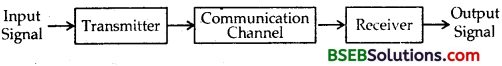Question 49.
Define a signal.
It is defined as a single valued function of time which conveys the information.Question 50.
Define phase modulation.
It is defined as the process of producing modulated wave such that the magnitude of the its phase angle varies in accordance with the amplitude of the modulating wave keeping amplitude and frequency same as that of the carrier wave.

Question 1.
Name the various Analog Communication Systems.
The following are the various analog communication systems:

1. Telegraphy
2. Telephony
4. Television network
6. Teleprinting
7. Telex.

Question 2.
Name the various digital communication systems.
The following are the various digital communication systems:

1. Fax
2. Mobile telephone network
3. e-mail (Electronic mail)
4. Tele conferencing
5. Telemetry
6. Communication satellites
7. Global positioning system (G.P.S.).

Question 3.
Enlist some merits and demerits of digital communication systems over analog communication systems. .
Merits:

1. It has a common format for encoding different kinds of message signals (like speech signal, video signal, computer data) for the transmission purpose.
2. There is an improved security of message. .
3. It has increased immunity to noise and external interference.
4. There is flexibility in configuring digital communication systems.

Demerits:

1. They have increased transmission bandwidth.
2. They have increased system complexibility.

Question 4.
Explain why modulation is required for transmitting audio signals over long distances?
Audio signals cannot be transmitted over long distances d ue to the following reasons :
(a) Size of antenna or aerial: For efficient transmission and reception, the transmitting and receiving antenna must have size comparable to the wavelength of the audio signal and atleast equal to quarter of the signal (= $$\left(=\frac{\lambda}{4}\right)$$) so that time variation of the signal is properly sensed by the antenna. For 15 kHz frequency, λ = $$\frac{\mathrm{c}}{\mathrm{v}}$$ = $$\frac{3 \times 10^{8}}{15 \times 10^{3}}$$ = 2 × 104 m. Thus antenna length must be $$\frac{\lambda}{4}$$ = 5000 m. To set up a vertical antenna of this size is practically impossible.

(b) The energy radiated from an antenna is practically zero when the frequency of the signal to be transmitted is below 15 kHz. Thus direct transmission of audio signals is not possible. We know that power radiated from a linear antenna of length l is $$P \propto \frac{1}{l^{2}}$$ . As high powers are needed for good transmission, l must be small for which λ should be small or v must be high.

(c) Mixing up of signals from different transmitters: The audio signals from different transmitting stations if are transmitted simultaneously then they get mixed up and there is no way to distinguish between them. Thus audio signals cannot be transmitted directly.

These difficulties can be overcome if the signals are transmitted at high frequencies. This is achieved by the process of modulation e.g. when a carrier wave of 1 MHz is used for transmission of audio signal, the antenna required will be of length 75 m only which can be easily set up.

Also the energy radiated from the antenna for a radio signal of frequency 1 MHz is quite appreciable and signal can travel upto a large distance before it dies out. The problem of mixing up of the signals from different transmitting stations is overcome by allocating different frequencies of the carrier waves to different transmitting stations.Question 5.
(i) FM transmission is highly efficient compared to AM transmission because the amplitude and hence power of FM wave is constant.

(ii) FM reception is almost immune to noise as compared to AM reception. Noise is a form of amplitude variations in the transmitted signal. In FM noise can be reduced by increasing the frequency deviation or by making use of amplitude limiters which is hot available in the AM wave.

(iii) FM transmission can be used for the stereo sound transmission due to large number of side bands which depend on modulation index (mf).
(iv) With the use of space wave in FM broadcasts, radius of operation is limited to slightly more than the line of sight transmission which reduces the chances of adjacent channel interference.

(i) The bandwidth (i.e., Channel Width) in FM transmission is about 10 times as large as that needed in AM transmission. So much wider frequency channel is required in FM transmission.

(ii) As FM reception is limited to line of sight, the area of reception of FM is much smaller than that of AM.

(iii) FM transmitting and receiving equipments are very complex as compared to those used in AM transmission.

Question 6.
What are advantages of digital communication?
(i) The digital signals are easy to receive. What all the receiver
needs to do is to detect whether a pulse is high or low.

(ii) AM and FM signals become corrupted over much short distances as compared to digital signals, so AM/FM signals cannot be reproduced faithfully. But in digital signals, the original signal can be reproduced accurately as long as receiver can distinguish between high and low.

(iii) The digital signals are not distored by the noise. The noise may change die shape of the pulses and not the pattern of the pulses that carries information in these signals.

(iv) Once AM/FM signal is transmitted, any one with a suitable receiver can listen in, but the digital signals can be coded so that only the person, they are intended for can receive them.

(v) The signals lose power as they travel. The digital signal can be cleaned up to restore quality and amplified at different stages by regenerators to restore the power.

(vi) The digital signals can be stored in the absence of the person at the receiving end but AM or FM signals can be received only at the time they are transmitted as AM or FM transmission constitute ‘real time systems’.

(vii) The digital signals can be stored as digital data or used to produce a display on a computer monitor or converted back into analog signal to derive a loudspeaker.

Question 7.
Explain the modes in which modems can be used.
Ans .
The following are the three modes of using modems :
(a) Simplex mode: It is the mode of using a modem in which data signals can be transmitted between the transmitter and receiver in only one direction.
(b) Half-duplex: It is the mode of using modem in which data signals can be transmitted between the transmitter and the receiver in both direction, but only in one direction at a time.
(c) Full-duplex: It is the mode of using a modem, in which data signals can be transmitted between the transmitter and the receiver in both directions at the same time.

Question 8.
Give a brief description of various elements required to transmit and receive back an audio signal.

1. The radio frequency oscillator generates radio frequency waves called carrier waves.
2. A microphone converts the sound waves into electrical waves i. e., audio signals.
3. The modulator mixes up the audio signal with the carrier waves and feeds the modulated waves to the transmitter.
4. The transmitting antenna radiates the modulated waves into the space.
5. The transmitted signal is intercepted by the receiving antenna.
6. The detector is, used to demodulate i.e., sepate out the audio signal from the modulated waves.
7. The loudspeaker converts the demodulated audio signal back into the sound waves.Question 9.
What is the shape of carrier in pulse modulation? Mention the types of pulse modulation in brief.
The carrier in pulse modulation consists of pulse train. It is no longer a continuous sinusoidal wave. In this modulation, some parameters of the carrier is varied in accordance with the instantaneous value of modulating signal.

Pulse modulation is of two types :
(a) Pulse amplitude modulation: The amplitude of the pulses of the carrier pulse train is changed in accordance with the instantaneous value of modulating signal.

(b) Pulse time Modulation: In this type ,of time modulation, timing of the pulses of the carrier, pulse train is canged in accordance with the instantaneous value of die modulating signal.
PTM is of following two types :-
(i) Pulse Width Modulation (PWM)—The pulse width of carrier pulse train is varied in accordance with the instantaneous value of the modulating signal. It is also called Pulse duration modulation (PDM) or Pulse length modulation (PLM).
(ii) Pulse Position Modulation (PPM)—In this modulation type, the position of the pulses of the carrier wave train is varied
in accordance with the instantaneous value of the modulating signal.

Question 10.
Define pulse code modulation (PCM). How an analog signal is pulse code modulated?
It is the process of obtaining completely digital modulation. The pulse amplitude, pulse width and pulse position modulations are not completely digital as die amplitude, width and position of die pulses transmitted vary continuously in accordance with the variations in the base band signal. The PAM signals are used to obtain PCM signals. The analog signal is pulse code modulated by using :
(a) Sampling: In this process the amplitude of analog signal is measured several times during each cycle of the signal.
(b) Quantisation: The amplitude of the analog voltage signal is divided into a large no. of levels called quantisation levels.
(c) Coding: The quantised pulses are digitised according to some code called coding. The most popular code being die binary code.

Question 11.
Explain in brief the various regions of earth’s atmosphere.
(a) Troposphere: It extends from earth’s surface upto 12 km. Its density varies from 1 kg m-3 at the surface of earth to 0.1 kg-3 m at the top of this layer.

(b) Stratosphere: It extends from 12 km from the surface of earth upto 50 km arid it forms practically the clear sky. The density of atmosphere in this layer varies from 0.1 kg m-3 to 10-3 kg m-3 and die temperature changes from 220 K to 280 K. In the lower region from 15 km to 30 km, there is ozone layer which results from the dissociation of oxygen by solar UV radiation. This layer absorbs most of the UV radiations coming from sun and so it protects the life on earth from its harmful effects.

(c) Mesosphere: It extends from 50 to 80 km from the earth’s surface. Density varies from 10-3 kg m-3 to 10-5 kg m-3 and temperature from 290 K to 180 K.

(d) Ionosphere: It extends from 80 km to 400 km from earth’s surface and it is the outermost part of the atmosphere. It receives maximum energy from thesun. As we go up, the temperature increases from 180 K to 700 K with altitude and density varies from 10-5 to 10~10 kg m-3.

Question 12.
Explain how Ground Wave propagation of radiowaves from transmitting antenna to receiving antenna takes place?
In amplitude modulated communication, the ground wave propagation takes place in following two ways:
(a) Surface wave propagation: When the radio waves from the transmitting antenna propagate along the surface of earth so as to reach the receiving antenna, the wave propagation is called so.
All amplitude modulated radio signals received in day time propagate by means of surface waves. Ground wave propagation for signals of very high frequency (such as TV and FM signals) is not used because at high frequency, the ground wave signal is reduced in strength by absorption to such an extent that it virtually becomes useless beyond an area of a few kms around the transmitting antenna.

(b) Space wave propagation: It is defined as the radiowave propagation from the transmitting antenna to the receiving antenna either directly or after reflection from the ground or in troposphere.
TV and FM signals can be transmitted by this method.
The maximum range of ground wave propagation depends on:
(ii) power of the transmitter.
The groundwaves propagation is suitable for low and medium frequency i.e., upto 2 MHz only. So it is also called medium wave propagation while space wave propagation is from 30 MHz to 300 MHz.

Question 13.
Write a short note on sky wave propagation.
It is defined as the radiowave propagation from the transmitting to receiving antenna after reflection in the ionosphere. The sky waves are the radiowaves of frequency between 2 MHz to 30 MHz. The sky waves are of practical importance at medium and high frequencies i.e., at medium waves and short waves for very long distance radio communication. The sky waves propagation is also called ionosphere propagation, since the sky waves reach the receiver after reflection from the ionosphere and they cover distances upto 400 km.Question 14.
Define the following:
(a) Critical frequency.
(b) Maximum usable frequency (MUF).
(c) Skip distance
(e) Refractive index.
(a) It is defined as the highest frequency of radio wave which when sent straight towards the layer of ionosphere gets reflected from ionosphere and returns to the earth. It is denoted by vc.
The radiowaves having frequency more than critical frequency will not be reflected by the ionosphere. The critical frequency of a sky wave for reflection from a layer of atmosphere is given by
vc = $$\mathrm{a}\left(\mathrm{N}_{\max }\right)^{\frac{1}{2}}$$
where Nmax = Number density of electrons/m3.
(b) It is the highest frequency of radio waves which when sent at some angle towards the ionosphere gets reflected from that and returns to the earth.
MUF = $$\frac{v_{c}}{\cos i}$$ = vc sec i
where i is the angle between normal and the direction of incidence of waves.
(c) It is the smallest distance from a transmitter along the earth’s surface which a sky wave of a fixed frequency but not more than critical frequency is sent to the earth.where h = height of reflecting layer of atmosphere.
vmax= maximum frequency of e.m. waves.
vc = critical frequency for that layer of atmosphere.
(d) It is defined as the change in the strength of a signal at a receiver due to interference of waves. Fading is more at high frequencies.
(e) n of a layer in sky wave propagation is given bywhere N = no. density of electrons m-3
v = frequency of e.m. waves in kHz.

Question 15.
Why sky wave propagation of em waves cannot be used for TV transmission? Suggest two methods by which range of TV transmission can be increased.
Sky wave propagation deals with amplitude modulated radio waves having frequency 1500 kHz to 40 MHz reflected by ionosphere of earth. TV transmission deals with frequency range of 80 MHz to 200 MHz. Their communication via sky waves is not possible because they are not reflected back by ionosphere. Range of TV transmission can be increased by (i) using satellite, (ii) using tall receiver antennas.

Question 1.
Calculate the height of the transmitting antenna and the population covered by it.
Let h be the height of the .T.V. transmitting antenna PQ located at a point P on earth’s surface. The signals transmitted from the antenna can be received with in a circle of radius QS or QT on earth’s surface.
Let R be the radius of earth. If d be the radius of the circle upto which the transmission can be received, thenAlso we know that as R >> d, so SQ or QT are tangent to SO or TO respectively or AQSO is a right angled triangle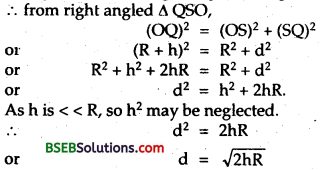If A be the covered area by the T. V. signal, then
A = πd2 = π. 2hR
Let σ be the population density, then population covered
= population density × Area covered.
= σ. A = σ. π. 2hr.

Numerical Problems

Question 1.
Calculate the bit rate for a signal which has a sampling rate of 8 kHz using 16 quantisation levels.
Let n = no. of bits per sample, then
no. of quantisation levels = 2n
No. of quantisation levels = 16 (Given)
= 24
∴ 2n = 24
or n = 4
bit rate = ?,
Here sampling rate = 8000 Hz.
We know that bit rate = sampling rate × no. of bits per sample
= 8000 × 4 = 32,000 bits s-1.Question 2.
In an amplitude modulator, the tank circuit consists of a coil of inductance 3.6 mH and a capacitor of capacitance 2.5 pF. If an audio signal of frequency 15 kHz modulates the carrier generated by the tank circuit, then find the frequencies of the side bands.
Here, L = self inductance of tank circuit = 3.6 mH
= 3.6 × 10-3 H
C = Capacitance of capacitor in tank circuit = 2.5 pF
= 2.5 × 10-12 F.
fm = frequency of modulating wave = 15 kHz = 15 × 103 Hz.
Let fc = frequency of carrier generated by the tank circuit.= 1.678 × 106 Hz = 1678 × 103 Hz = 1678 kHz.
frequency of side bands is given by fc ± fm.
frequency of the upper side band is fc + fm = (1678 + 15) kHz = 1693 kHz.
and frequency of the lower side band is given by
fc – fm = (1678-15) kHz
= 1663 kHz.

Question 3.
An audio signal of 2.8 kHz modulates a carrier of frequency 90 MHz producing a frequency deviation of 84 kHz. Find (a) frequency modulation index, (b) frequency range of the modulated wave.
Here, fc = frequency of carrier = 90 MHz = 90 × 103 kHz.
fm = frequency of modulating wave = 2.8 kHz
δ = frequency deviation = 84 kHz.
(a) mf = frequency modulation index = ?
We know that, mf = $$\frac{\delta}{\mathrm{f}_{\mathrm{m}}}$$ = $$\frac{84}{2.8}$$ = 30
(b) Frequency range of modulated wave = Frequency of side bands
(fc ± fm) = (fc – fm) to (fc + fm)
∴ (fc – fm) to fc + fm
= (90,000 – 2.8) kHz to (90,000 + 2.8) kHz.
= 89997.2 kHz to 90002.8 kHz
= 89.997 × 103 kHz to 90.003 × 103 kHz.
= 89.997 MHz to 90.003 MHz.

Question 4.
An audio signal of amplitude (a) equal to (b) 1.5 times the carrier amplitude is employed in amplitude modulation. Find the modulation index in each case.
Em = amplitude of audio signal.
Ec = amplitude of carrier signal.
(a) Em = Ec. (Given),
∴ ma = modulation index = ?
∴ Emax = Ec + Em = Ec + Ec = 2 Ec.
and Emin = Ec – Em = Ec = Ec = 0.
∴ Using the relation,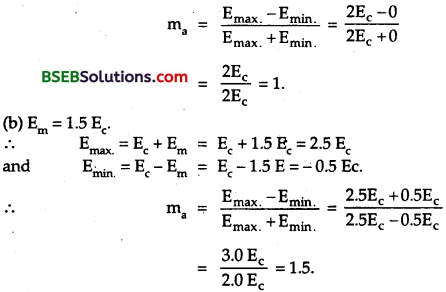Question 5.
A transmitter transmits a power of 20 kW, when modulation is 50%. Calculate power of the carrier wave.
Here Pt = power of transmitter = total power in the modulated wave = 20 kW.
Pc = Power of unmodulated carrier wave = ?
m = modulation index = 50% = $$\frac{50}{1oo}$$ = $$\frac{1}{2}$$
Using the relation,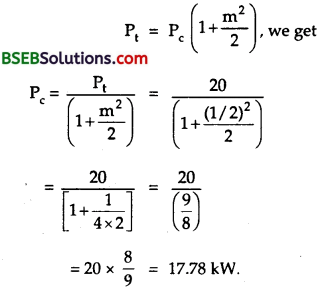Question 6.
Calculate the length of the dipole antenna for a carrier wave of frequency 3 × 108 Hz.
Here, v = frequency of carrier wave = 3 × 108 Hz.
l = length of antenna = ?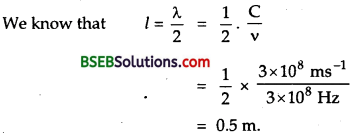Question 7.
The carrier frequency of a station is 50 MHz. A resistor of 10 KΩ and a capacitor of 10 pF are available in the detector circuit. Is it good enough for detection?
Here, R = 10 KΩ = 10 × 103 Ω = 104
C = Capacitance of capacitor = 10 pF = 10 × 10-12 F = 10-11 F.
fc = frequency of carrier wave = 50 MHz = 50 × 106 Hz = 5 × 107 Hz.
∴ Time constant of the circuit is given by
τ = RC = 104 × 10-11 = 10-7 s. …..(i)
For demodulation i.e. for detection,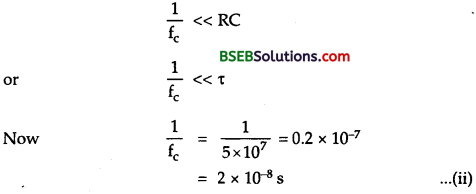It is dear from (i) and (ii) that $$\frac{1}{\mathrm{f}_{\mathrm{c}}}$$ << τ i.e., the condition of detection is satisfied, so the circuit is good enough for detection.

Question 8.
A T.V. tower has a height 100 m. What is the population density around the T. V. tower if the total population covered is 50 lakh? Radius of earth – 6.4 × 106 m.
Here, h = height of tower = 100 m 50
population covered = lakh = 50 × 105
R = radius of earth = 6.4 × 106 m.
δ = Population density = ?
We know that,Question 9.
A T.V. transmission tower at a particular station has a height of 160 m.
(a) What is its coverage range?
(b) How much population is covered by transmission, if the average population density around the tower is 1200 km-2?
(c) By how much the height of tower be increased to double its coverage range? Given radius of earth = 6400 km.
Here, h = 160 m
R = 6400 km = 6.4 x106 m.
(a) d = coverage range = ?
We know that d = >$$\sqrt{2 \mathrm{hR}}$$ = $$\sqrt{2 \times 160 \times 6.4 \times 10^{6}}$$
= 45255 m = 45.26 × 103 m = 45.26 km.

(b) Population covered = ?
Here, δ = population density = 1200 km-2
∴ Population covered = δ × π d2
= 1200 km-2 × $$\frac{22}{7}$$ × (45.26 km)-2
= 77.24 × 105
= 77.24 lakh.

(c) Let d’ be the new coverage range.
∴ d’ = 2d
Let h’ = height of tower required for a coverage up to d’
∴ h’- h = ?
∴ d’ = $$\sqrt{2 \mathrm{~h}^{\prime} R}$$ = 2d = 2 $$\sqrt{2 \mathrm{hR}}$$
or 2h’R = 42 hR
or h’ = 4h = 4 × 160 m = 640 m
increase in height of tower = h’ – h
= 640 – 160 = 480 m.Question 10.
Calculate the time delay between the reception of the ground wave and the sky wave for a receiver at a distance of 200 km from the transmitter, given that the reflecting ionosphere layer is effectively at a height of 100 km.
Here, AB = distance between transmitter and receiver = 200 km = 2 × 105 m.
Height of the ionosphere layer,
CN = 100 km = 105 m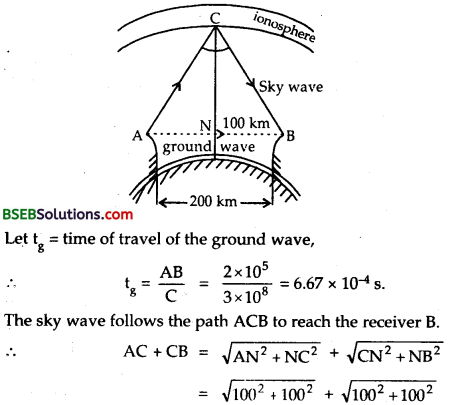= 100 √2 +100 √2
= 200 √2 = 200 × 1.414 = 282.800 km
= 283 km = 2.83 × 105 m.
Let ts be the time of travel of the sky wave, then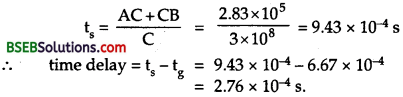Question 11.
In a radio receiver, the short-wave and medium-wave stations are turned by using the same capacitor but coils of different inductance. Explain, why the inductance in the former case is less than that in the latter case?
Let λs and λm be the wavelength of short-wave station and medium-wave station.
If vs and vm be the corresponding frequencies of short wave and medium-wave signals, thenNow Let C = capacitance of the capacitor.
If Lm and Ls be the inductance of the medium-wave and short wave coils respectively.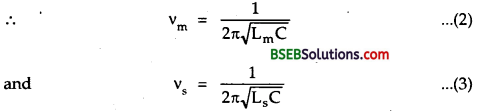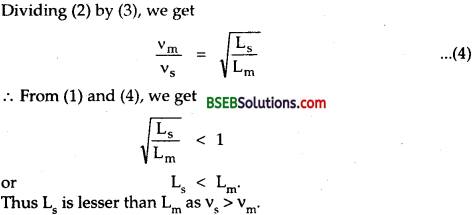Question 12.
A radio can tune to any station in the 7.5 MHz to 12 MHz band. What is the corresponding wavelength band?
Here, v1 = 7.5 MHz = 7.5 × 106 Hz.
and v2 = 12 MHz = 12 × 106 Hz.
If λ1 and λ2 be the corresponding wavelengths, thenThus wavelength band corresponding to the given frequency band is 25 m to 40 m.

Question 13.
If the maximum values of signal and carrier waves are 4V and 5V respectively, then find :
(a) the maximum and the minimum value of the modulated amplitude in volt.
(b) the percentage of modulation.
Here, Vs = 4V, Vc = 5V.
(a) (i) maximum modulated amplitude or maximum modulated voltage
= Vc + Vs = 5 +4 = 9 V
(ii) minimum modulated voltage = Vc – Vs = 5 – 4 = 1V.

(b) m % = percentage of modulation = ?
We know that
μ or m = $$\frac{\mathrm{V}_{\mathrm{s}}}{\mathrm{V}_{\mathrm{c}}}$$ × 100 = $$\frac{4}{5}$$ × 100 = 80%.

Question 14.
Frequencies higher than 10 MHz are found not to be reflected by the ionosphere on a particular day at a place. Calculate the maximum electron density of the ionosphere.
Critical frequencies vc = 10 MHz = 10 × 106 = 107 Hz.
Suppose Nmax is maximum electron density of ionosphere. Then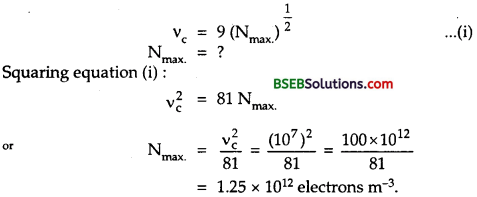Question 15.
A radar has a power of 1 kW and is operating at a frequency of 10 GHz. It is located on a steep mountain top of height 600 m. What is the maximum distance up to which it can detect an object located on the surrounding earth’s surface?
Given, h = 600 m
We know, R = 6400 km = 64 × 105 m
Coverage range, d = $$\sqrt{2 \mathrm{Rh}}$$
= $$\sqrt{2 \times 64 \times 10^{5} \times 600}$$
= 71520 m
= 71.52 km.Question 16.
In previous question, suppose the antenna at the mountain top is 100 m and the Radar power is double to 2 kW. What ) is the maximum distance which it can cover now?
Given h = 100 m
We know that R = Radius of earth = 6400 km
= 6400 × 1000 m = 64 × 105 m
Coverage range d = $$\sqrt{2 \mathrm{Rh}}$$
= $$\sqrt{2 \times 64 \times 10^{5} \times 100}$$
= 51.36 × 105 m = 51.36 km.

Question 17.
On a particular day, the maximum frequency reflected from the ionosphere is 10 MHz. On another day, it was found to increase to 11 MHz. Calculate the ratio of the maximum electron densities of the ionosphere on the two days. Point out a plausible explanation for this.
Maximum frequency reflected from ionosphere
= 10 MHz = 10 × 106 = 107 Hz.
∴ v1(c) = 107 Hz
Similarly v1(c) = 11 MHz = 11 × 106 = 1.1 × 107 Hz
Maximum electron densities corresponding to v1(c) and v2(c) are N1max. and N1max. respectively.Fill in the Blanks

Question 1
……………. is a device which converts energy from one form to another.
Transducer.

Question 2
……………. separates out the audio signal from the modulated signal.
Demodulator.

Question 3.
Marconi antenna has length while Hertz antenna has a length equal to …………….
$$\frac{λ}{4}$$ , $$\frac{λ}{2}$$

Question 4.
The length of antenna required to transmit a radio signal of frequency 1 MHz is about …………….
75 m.

Question 5.
The bandwidth of a complex audio signal is equal to ……………. of the audio signal.
Twice the maximum frequency.

Question 6.
In-frequency modulated signal the informatibn is contained in the ……………. and the bandwidth is …………….
side bands, 2n × frequency of modulating signal where n is the number of significant side band pairs.

Question 7.
To achieve pulse code modulation an analog signal ……………., ……………. and then coded.
sampled, quantised.

Question 8.
A digital signal is described by the …………….
bit rate i.e., number of binary digits (bits) per second.

Question 9.
The electromagnetic waves of radio frequencies are reflected by ……………. and this effect is used for ……………. round the curved surface of earth.

Question 10.
The bandwidth for AM radio station is ……………. and for an FM radio station, the bandwidth is …………….
10 kHz, 150 kHz.

Question 11.
The modulation index of an AM wave lies between …………….
0 and 1.

Question 12.
The rate at which a modem can transmit and receive data is expressed as …………….
BPS (bits per second).

Question 13
……………. is preferred for high fidelity reception.
Frequency modulation.

Question 14.
Bit rate = ……………. × number of bits per sample.
Sampling rate.

Question 15
……………. are the relevant waves in telecommunication.
Microwaves.

Question 16.
We use short wave bands only for long distance radio broadcast because …………….
Ionosphere easily reflects the waves in this band.

Question 17
……………. uses carrier signals having frequencies in the range 1012 Hz to 1016 Hz.
Optical communication.Question 18.
The electromagnetic wave of frequency 120 MHz belongs to …………….
Frequency modulated band.

Question 19.
The essential condition for demodulation is that the time period of high frequency carrier wave must be …………….
or $$\frac{1}{\mathrm{f}_{\mathrm{c}}}<<\mathrm{RC}$$.
i.e., m = $$\frac{\mathbf{E}_{\mathrm{m}}}{\mathrm{E}_{\mathrm{c}}}$$ = $$\frac{V_{m}}{V_{c}}$$.